[BACK]
 Intelligent Automation & Soft ComputingDOI:10.32604/iasc.2021.018516Article

Prediction of the Corrosion Rate of Al–Si Alloys Using Optimal Regression Methods

1Industrial Engineering Department, College of Engineering, Taif University, Taif, 21944, Saudi Arabia
2Electrical Engineering Department, College of Engineering, Taif University, Taif, 21944, Saudi Arabia
3Mechanical Engineering Department, College of Engineering, Taif University, Taif, 21944, Saudi Arabia
*Corresponding Author: D. Saber. Email: dselsayed@tu.edu.sa
Received: 11 March 2021; Accepted: 13 April 2021

Abstract: In this study, optimal regression learner methods were used to predict the corrosion behavior of aluminum–silicon alloys (Al–Si) with various Si ratios in different media. Al–Si alloys with 0, 1%, 8%, 11.2%, and 15% Si were tested in different media with different pH values at different stirring speeds (0, 300, 600, 750, 900, 1050, and 1200 rpm). Corrosion behavior was evaluated via electrochemical potentiodynamic test. The corrosion rates (CRs) obtained from the corrosion tests were utilized in the formation of datasets of various machine regression learner optimization (MRLO) methods, namely, decision tree, support vector machine, Gaussian process regression, and ensemble method. Stirring speeds, solution pH, and Si ratio were adopted as inputs, whereas the CRs were employed as the outputs. These parameters were applied to build optimal models of the four MRLO methods. The regression learner methods were implemented and conducted in 2020b MATLAB/software regression learner toolbox. The MRLO methods were validated by comparing them with an artificial neural network (ANN) model. Experimental results showed that the CR of the Al–Si alloys increased with the increase in stirring speeds. The highest CR was recorded at pH 3.5. Moreover, the addition of Si to pure Al as a hypoeutectic alloy (1% and 8% Si) or a hypereutectic alloy (15% Si) improved the CR of pure Al. The CR in the solution containing only Al2O3 particles with pH 7.75 was smaller compared with that of the solution containing H2SO4. The Gaussian process regression model had the highest CR prediction accuracy with the lowest minimum mean square error (0.000446607). The results demonstrated that the proposed GPR model was more effective than the ANN model.

Keywords: Al–Si alloys; corrosion rate; stirring speed; prediction model; machine regression learning optimization methods; artificial neural network

1  Introduction

Aluminum casting alloys are an essential part of the manufacturing of shaped castings, especially in the aerospace and automotive industries, owing to their favorable properties, such as low density, good formability, high strength-stiffness-to-weight ratio, and good corrosion resistance. Al–Si alloys are used to produce cylinder heads, cylinder blocks, crankshaft, and pistons . The Si content of Al–Si alloys determines not only their mechanical properties but also their corrosion resistance . Salih et al.  assessed the corrosion behavior of Al–Si alloys with different compositions in 0.1 M sodium tartrate, sulfate, and borate solutions. They found that an increase in pH decreased polarization resistance. Mazhar et al.  investigated the role of chloride in the pitting of some Al–Si alloys via electrochemical polarization and electrochemical impedance measurements. At neutral pH, corrosion current initially increased and then decreased with chloride ion concentrations. In another study by Mazhar et al. , they evaluated some Al–Si alloys in acidic and alkaline media. The Al–Si alloys corroded at a higher rate than pure Al in these media. In addition, they found that eutectic alloy had the highest corrosion rate (CR).

The microstructure of Al–Si alloys strongly corrosion affects resistance. The microstructure of Al–Si alloys is constructed from α–Al solid solution and various secondary phases, such as AlFeSi, AlFeSiMn, Mg2Si, and AlFeSiMg. The most common form of Al corrosion is pitting corrosion. Accordingly, the dependence of corrosion behavior on the microstructure of Al–Si alloys with different Si contents is currently being investigated. Although Si comprises a substantial volume fraction of most Al–Si alloys, its effect on the corrosion properties of Al–Si alloys is minimal because of the low corrosion current density that results from the high polarization of Si particles. The local cells formed by Fe and Si aid the pitting attack on the surface of Al–Si alloys in a conductive solution. The corrosion behavior of Al–Si alloys depends on the localized aggressive environment containing halide anions, which may break the passivated metal surface and lead to pitting [9,10].

New computational methods have been recently developed and introduced in various fields, including materials science. The neural network theory based on previously acquired data, that is, training set, is commonly used to test the success of a system by using test data. Results of artificial neural network (ANN) are in good agreement with experimental data. Moreover, ANN obtains additional useful data from small experimental databases. Thus, a trained neural network can achieve a very good performance. Results of experimental tests and those obtained by using neural networks are largely coincident . Corrosion features must be correctly predicted to efficiently control the progression of corrosion . ANNs are regarded as a solution to the attendant problems in predicting corrosion properties. Thike et al.  used a large dataset of atmospheric corrosion data of carbon steel compiled from several resources to train and test a multilayer backpropagation ANN model, as well as two conventional corrosion prediction models, namely, linear and Klinesmith models. Kenny et al.  established an ANN with linear and sigmoidal functions to predict the CRs of Al, low carbon steel, and Cu as a consequence of meteorological factors. Zhang et al.  assessed the atmospheric corrosion performance of bainite steel in exposed offshore platforms via ANN. Lo et al.  developed a regional forecasting model by using ANN to predict the atmospheric CR of Cu within general and coastal industrial zones in Taiwan. Li et al.  modeled the atmospheric corrosion behavior of Al alloys in 10 typical atmospheric corrosion test sites. Vera et al.  utilized several ANNs to predict the atmospheric CRs of Al, carbon steel, galvanized steel, and Cu. Willumeit et al.  demonstrated that ANN can predict well the corrosion properties of Mg alloys.

In the present work, the corrosion behavior of Al–Si alloys with various Si ratios in different media was assessed via electrochemical potentiodynamic test. The CRs obtained from the corrosion tests were used in the formation of datasets of four machine regression learner optimization (MRLO) methods, namely, decision tree (DT), support vector machine (SVM), ensemble method (EN), and Gaussian process regression (GPR), to predict the CRs. The four MRLO methods were conducted and implemented in 2020b MATLAB/software regression learner toolbox. Results showed that GPR had the best CR prediction accuracy. Finally, the proposed GPR model was validated, and its effectiveness was compared with that of an ANN model.

2  Experimental Procedure

Several castings of Al–Si alloys with various compositions were prepared via permanent die-casting. The chemical composition of the Al–Si alloys is shown in Tab. 1. In the corrosion test, the specimens were cut into circular disks with a diameter of 10 mm and a thickness of 5 mm. The specimens were mounted on a special acrylic mount. The specimens were prepared by grinding with 800 grit sandpaper. A small hole was made into which the electrical connection of the specimens were mounted in the corrosion cell.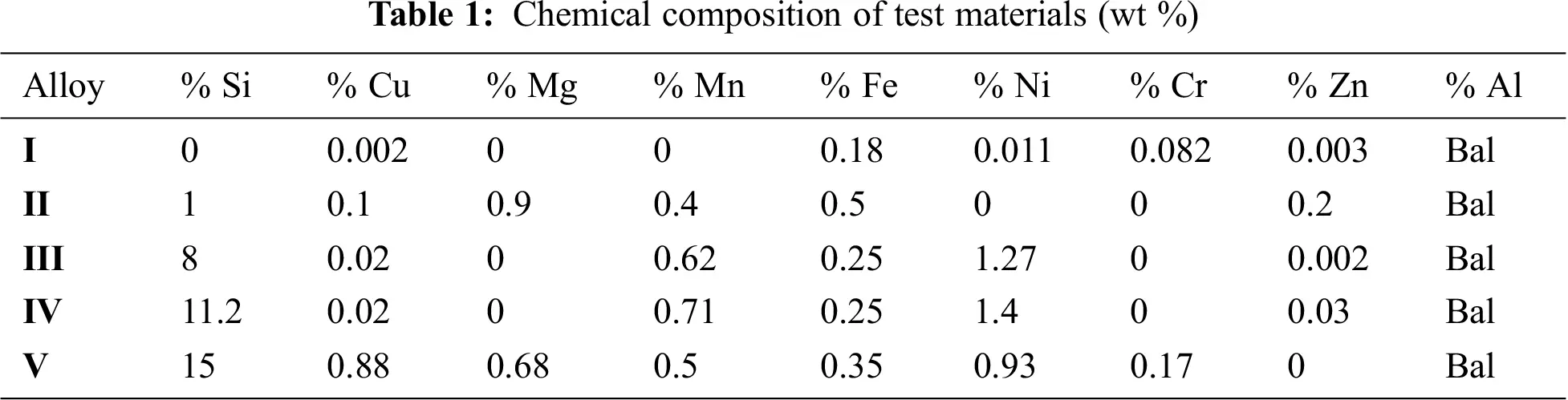Corrosion tests were conducted via potentiodynamic tests. The corrosion test consisted of a magnetic stirrer, a corrosion cell composed of the test specimens, a reference calomel electrode, and an auxiliary graphite electrode. These parts were immersed in a glass cylinder containing different solutions, as shown in Tab. 2. The potentiodynamic corrosion test was performed at stirring speeds of 0, 300, 600, 750, 900, 1050, and 1200 rpm. Electrochemical parameters were determined using a Minslberg potentiostat/galvanostat (PS6). The initial and final potential and the scanning rate were adjusted. The output of each run consisted of a polarization curve through which the corrosion parameters were determined via the Tafel extrapolation technique by using the software package supplied by the manufacturer.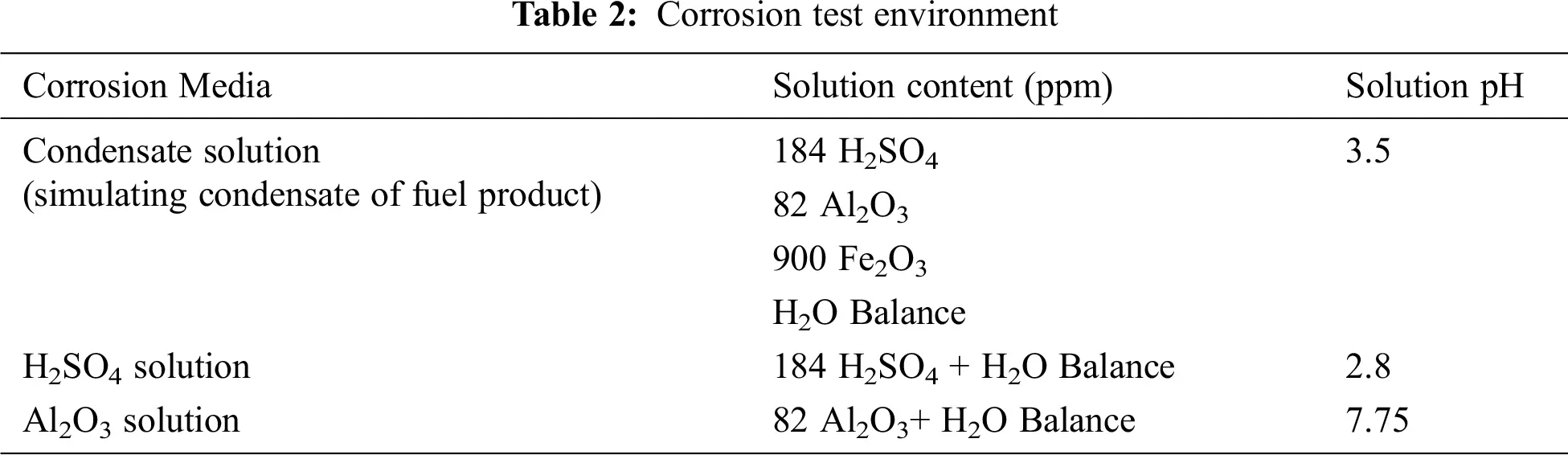3  Optimal Regression Learner Methods

The ability of the aforementioned MRLO methods in detecting the CR of the Al–Si alloys was evaluated. The MRLO methods involved four main regression optimization techniques: DT, SVM, GPR, and EN. Each regression optimization method has several sub-regression algorithms. For example, GPR is categorized into squared exponential, rational quadratic, squared exponential, exponential, Matern 3/2, and Matern 5/2. Various MRLO methods are generally used in different regression applications to determine the best optimization regression technique according to the training dataset. Stirring speeds, solution pH, and Si ratio (SR) were adopted as inputs, whereas the CRs were utilized as outputs. These parameters were employed to build optimal models of the MRLO methods. The MRLO methods were built using the 2020b MATLAB/software regression learner .

The training procedure can be summarized as follows:

1.    The dataset is divided into a training dataset (84 practical dataset samples) and a testing dataset (21 practical dataset samples).

2.    A validation approach is chosen. A cross/fold validation with five folds is used in the validation process during the training stage.

3.    A regression optimization technique is selected, and the number of training learners is identified.

4.    An optimization technique is selected, and the hyperparameters to use are determined.

5.    The regression model selected is trained.

6.    The regression model parameters selected are accessed.

7.    The selected regression model is exported.

8.    Steps 3 to 7 are repeated for all the other MRLO methods.

The optimal parameters of the MRLO methods were identified using different optimization techniques, such as grid search, Bayesian optimization (BO), and random search. The BO approach is the more general approach for optimization problems, and it is used for most machine learning regression techniques for parameter optimization determination . BO iteratively discovers the hyperparameter space, where a probabilistic model of approximation is built based on prior estimation. Finally, the probabilistic model is applied to estimate the optimal parameters by applying the probability values of its position and choosing the parameters related to peak probability . More details about the BO approach are presented in [22,23]. The main optimization selection parameters used during the training are listed in Tab. 3, whereas the optimal parameters of the MRLO methods are enumerated in Tab. 4.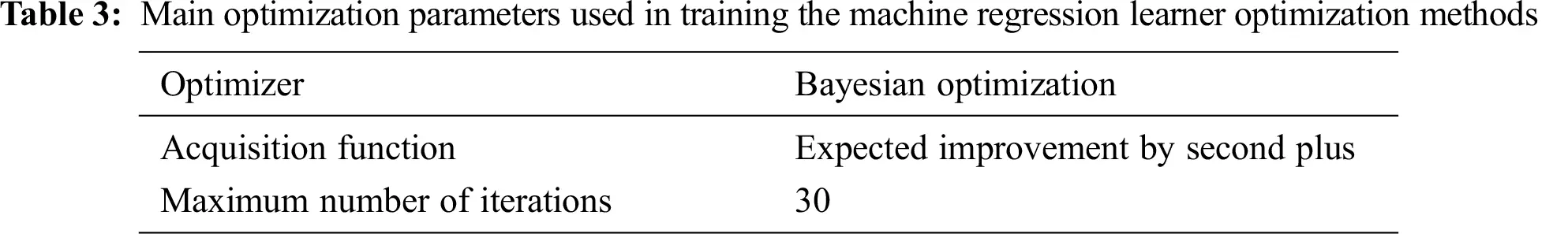Fig. 1 presents the minimum mean square error (MSE) of the different MRLO methods against the number of iterations during the optimization process based on the training dataset samples. GPR had the lowest MSE (0.000446607). MSE is estimated as follows:

MSE=1Ni=1N(OiPi)2 (1)

where N is the number of dataset samples; and Oi and Pi are the ith output and the MRLO predicted values, respectively.

Fig. 2 presents the predicted response versus the true response for the different MRLO methods during the training stage. GPR had the best prediction response.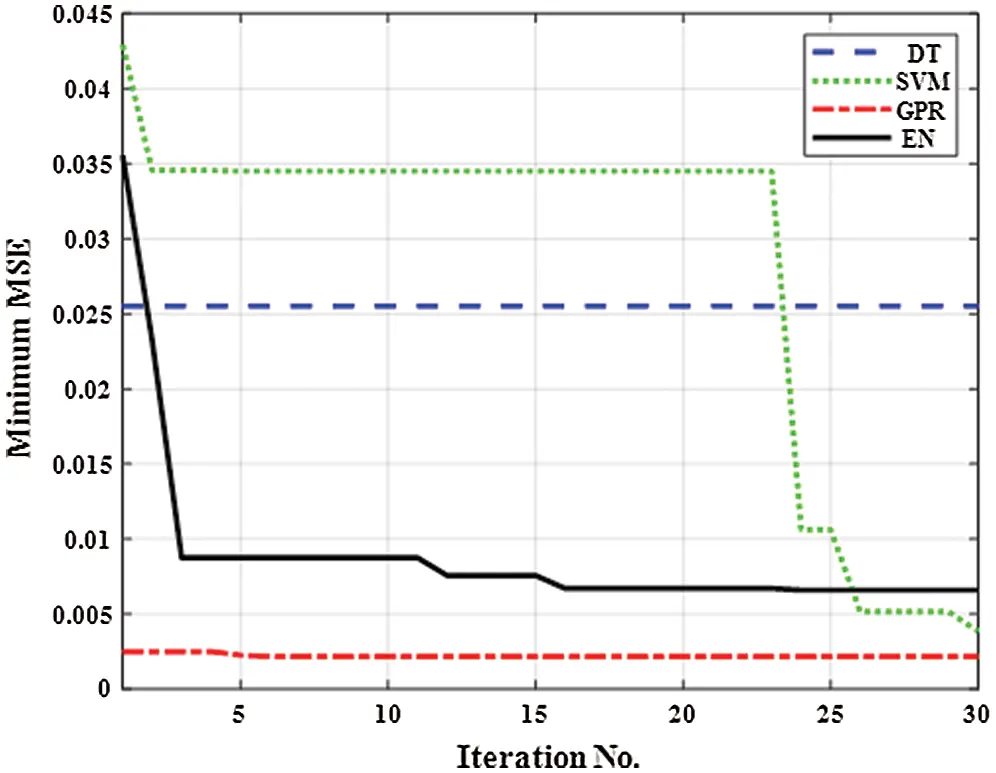Figure 1: Minimum mean square error of different machine regression learner optimization methods versus iteration number during the optimization process based on the training dataset samples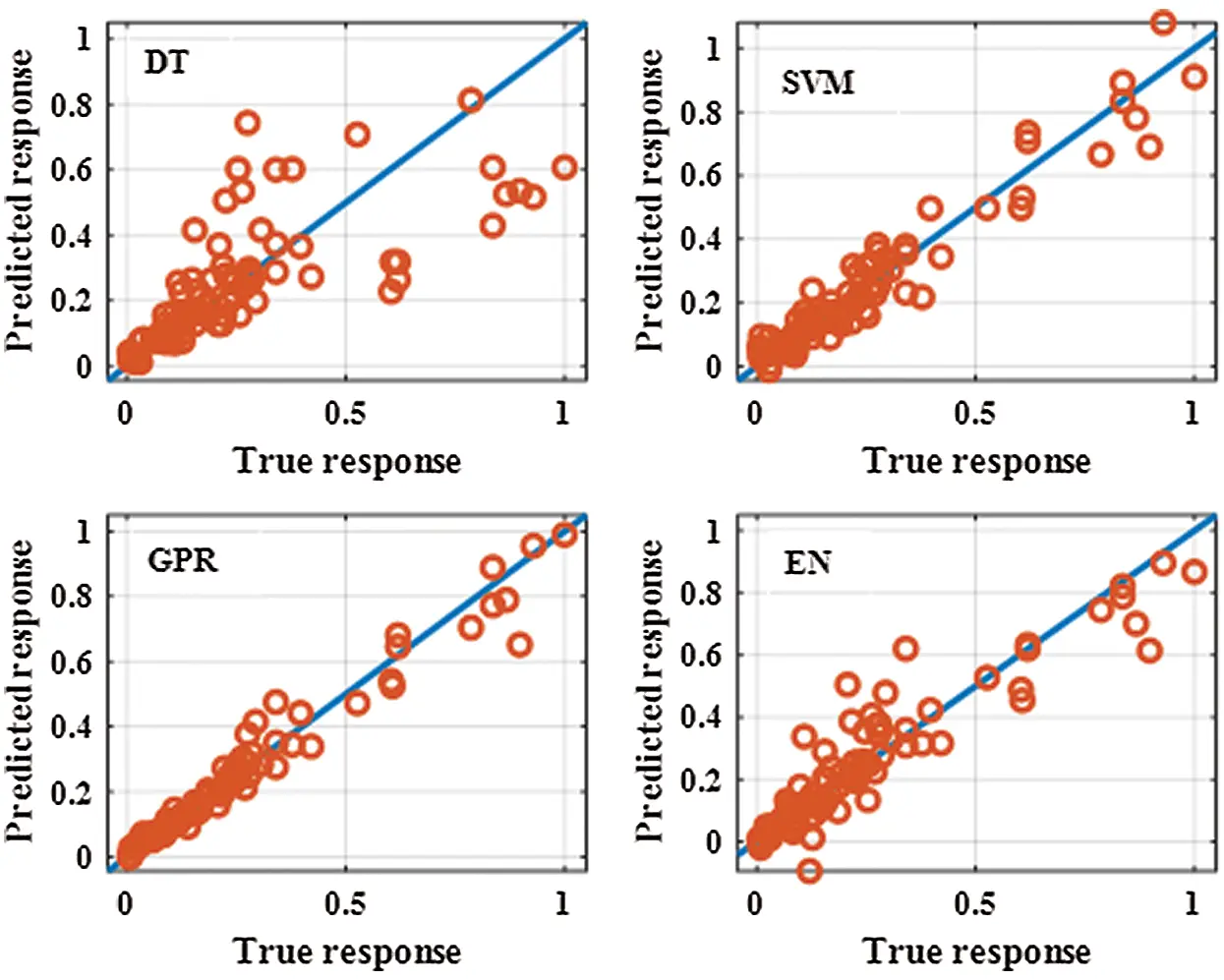Figure 2: Predicted response against true response for the different machine regression learner optimization methods during the training stage

4  Results and Discussion

4.1 Corrosion Behavior

The corrosion behavior of the selected Al–Si alloys in aqueous solution was complex. Results showed that corrosion behavior depended on solution stirring speed, pH, anion, and alloy composition [6,24]. As shown in Fig. 3, the CR of eutectic alloy (11.2% Si) was higher than that of both hypoeutectic alloy (1% Si and 8% Si) and hypereutectic alloy (15% Si) in different solution pH. This result was obtained probably because of the stabilization of the oxide layer due to the incorporation of elemental Al phase in the case of hypoeutectic alloys or elemental Si in the case of hypereutectic alloys. Moreover, the high CR of eutectic alloy could be attributed to its relatively fine-grained structure and high surface energy [7,8]. As shown in Fig. 3, the CR in simulated condensate solution with pH 3.5 was higher than that in other test solutions. The CR in the solution containing Al2O3 particles with pH 7.75 was very small compared with that of the solution containing H2SO4 and simulated condensate solution because of the neutral pH of the solution containing Al2O3 particles and the nonaggressive nature of the solution. Furthermore, the difference in CRs obtained in the existence of different anions was clarified by the chemical nature of corrosion reactions, which contained elemental anion species; these anions either caused the breakdown or the construction of protective reaction products on the surface . As shown in Fig. 4, the CRs markedly increased with increasing stirring speed. High rotation speeds were accompanied by high CRs, leading to the rapid removal of the passive film under the erosive action of Al2O3 and Fe2O3 particles. This result implied that the resulting unpassivated metal would have more opportunities to passivate per unit time; hence, current oscillation would increase the frequency. Two acting mechanisms were involved: first, metal dissolution due to both the electrochemical action of acidic solution and the mechanical action of erosive Al2O3 and Fe2O3 particles. Thus, the effects of Al2O3 and Fe2O3 particles in the solutions were greater under stirring conditions than those under stagnant conditions. The second acting mechanism was re-passivation due to the availability of sufficient amounts of oxygen; this re-passivation range depends on the available oxygen according to different stirring speeds .Figure 3: Effects of pH on the CR of Al–Si alloys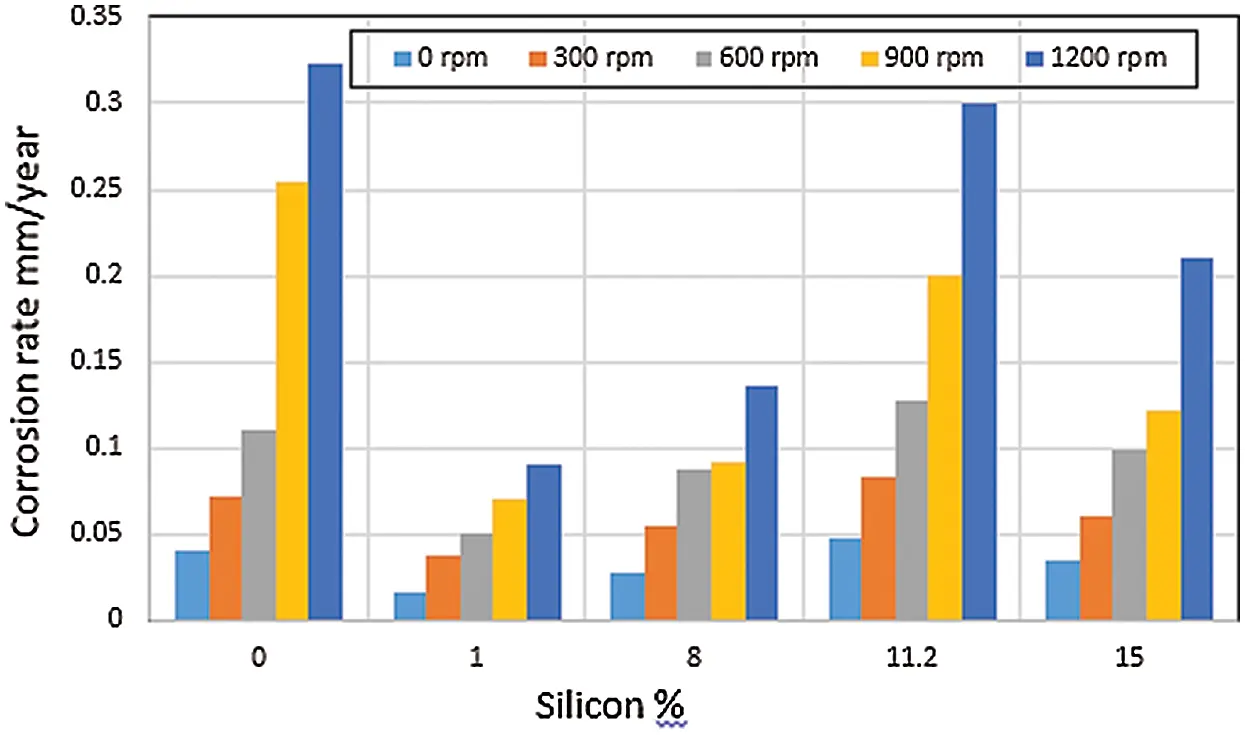Figure 4: Effects of stirring speed on the CR of Al–Si alloys

Scanning electron micrographs of the Al–Si alloys with different Si contents after the corrosion test in simulated condensate solution are presented in Fig. 5. Both pure Al and Al–Si alloys were clearly attacked by pitting corrosion. The severity of pitting corrosion depended on the percentage of Si in the alloy. Fig. 5a shows that pure Al had an extensive and intensive pitting. The size of the pits was substantially reduced by the addition of 8% Si (Fig. 5b). The attacked areas were mainly concentrated around some of the small Si sticks. Fig. 5c shows the microstructure of eutectic alloy (11.2% Si). It consisted of long Si sticks in the solid Al solution matrix. Given that this alloy was a eutectic alloy with a relatively fine-grained structure and had a high energy structure, it had numerous galvanic corrosion areas. The corrosion pits that formed on the surface of the eutectic alloy were large and indicated that a high CR occurred, in agreement with the results obtained in Figs. 3 and 4. As shown in Fig. 5d, the microstructure of the Al–Si alloy with 15% Si consisted of numerous flat plates and little stick-like Si particles distributed in the solid Al solution matrix. As indicated in Fig. 3d, this alloy suffered a low degree of attack. As can be concluded from Fig. 5, the addition of Si to pure Al as a hypoeutectic alloy or a hypereutectic alloy improved the CR of pure Al. By contrast, the presence of Si around the composition of the eutectic alloy led to severe corrosion.Figure 5: Microstructural examination of Al–Si alloys after the corrosion test in condensate solution under stagnant conditions (0 rpm) via scanning electron microscopy (a) Pure Al (b) 8% Si (c) 11.2% Si (d) 15% Si

4.2 Prediction of CR by the MRLO Methods

4.2.1 Prediction Performance of the MRLO Methods

The MRLO models for GPR, DT, SVM, and EN were used to predict the CR of 21 practical dataset samples. The prediction outputs of the four MRLO methods are summarized in Tab. 5. Results demonstrated that the different MRLO methods, especially GPR, had a good prediction performance.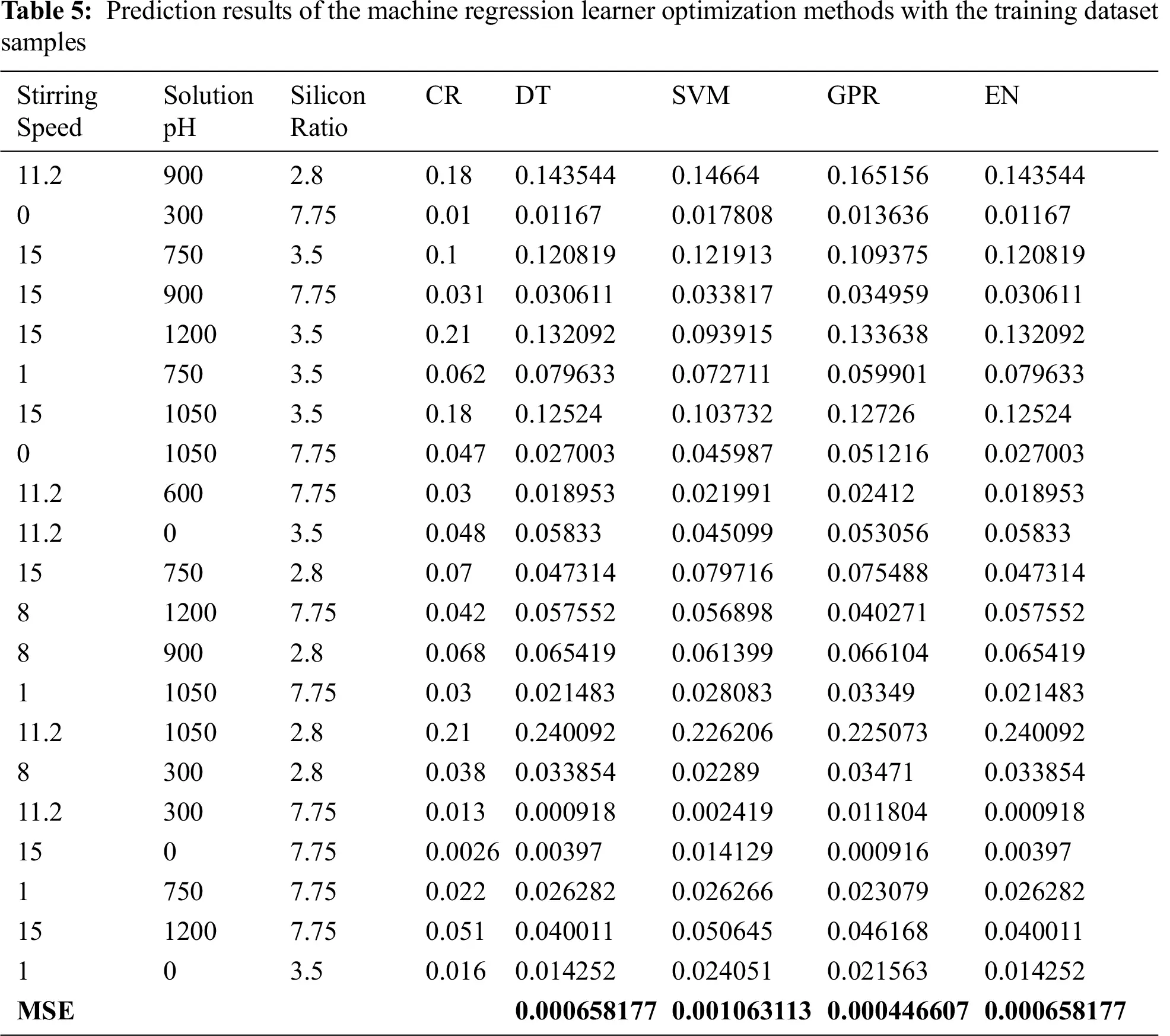4.2.2 Comparison of the MRLO Methods with ANN Method

ANN is widely used for regression and classification processes. ANN consists of three main layers, namely, an input layer, a hidden layer, and an output layer, as shown in Fig. 6. Each layer is composed of a number of neurons. The number of input layer is equal to the number of input features. Hidden layer neurons are selected to obtain the highest prediction accuracy, whereas output layer neurons are equal to the number of output variables . The relation between output m (Om) value and input features can be expressed as follows:

Om=G(i1nwimIibm), (2)

where G is the gain of nonlinear function used with the hidden layers, wim is the weight of ith input (Ii), and bm is the bias of output m. ANN has different types, among which the backpropagation type is the most widely used in regression processes .

The two main training algorithms for the training process of ANN are Levenberg–Marquardt (LM) and Bayesian regularization algorithms . In this work, the LM algorithm was utilized for the training process of the ANN model with different numbers of hidden layer neurons to determine the suitable number of neurons of the hidden layer. The training dataset was divided into three groups, namely, training (70%), testing (15%), and validation (15%) groups. Fig. 6 shows the MSE of the ANN model with the number of hidden layer neurons ranging from 1 to 100. The ANN model with a hidden layer with 14 neurons obtained the smallest MSE of 0.0241 (Fig. 7). Fig. 8 presents the regression of training, validation, testing, and all dataset samples with 14 hidden layer neurons; the regressions were 0.9909, 0.79726, 0.93789, and 0.96117, respectively. The ANN model obtained from the training process was employed to predict the CR with the test dataset samples. Tab. 6 presents a comparison between the proposed GPR and the ANN model with five samples from the testing dataset. The prediction results of GPR were slightly different from the actual CR, whereas the prediction results of the ANN model were substantially different from the actual CR. The overall MSE of the proposed GPR and ANN models for the testing dataset samples (21 samples) was 0.000446607 and 0.009293406, respectively. Results demonstrated that the proposed GPR model was more effective than the ANN model. In previous studies, the prediction results of the ANN model were compared not only with experimental results but also with the results of other models. Pintos et al.  proved that ANN-based methodology is better than a linear regression model and has a good agreement with known data for modeling atmospheric corrosion. Cai et al.  constructed two different ANN models to model the atmospheric corrosion of carbon steel and zinc. Thike et al.  compared the evaluation metrics of three models. They reported that the RMSE of the linear model (0.1356) was the highest, and ANN had a slightly lower RMSE value (0.1011) than the Klinesmith model. According to the results of the comparison of the evaluation metrics for the three models for new data, the ANN model exhibits a better performance than conventional models in predicting the atmospheric corrosion of carbon steel. Moreover, the Klinesmith model provides better prediction results than the linear model.Figure 6: Construction configuration of artificial neural network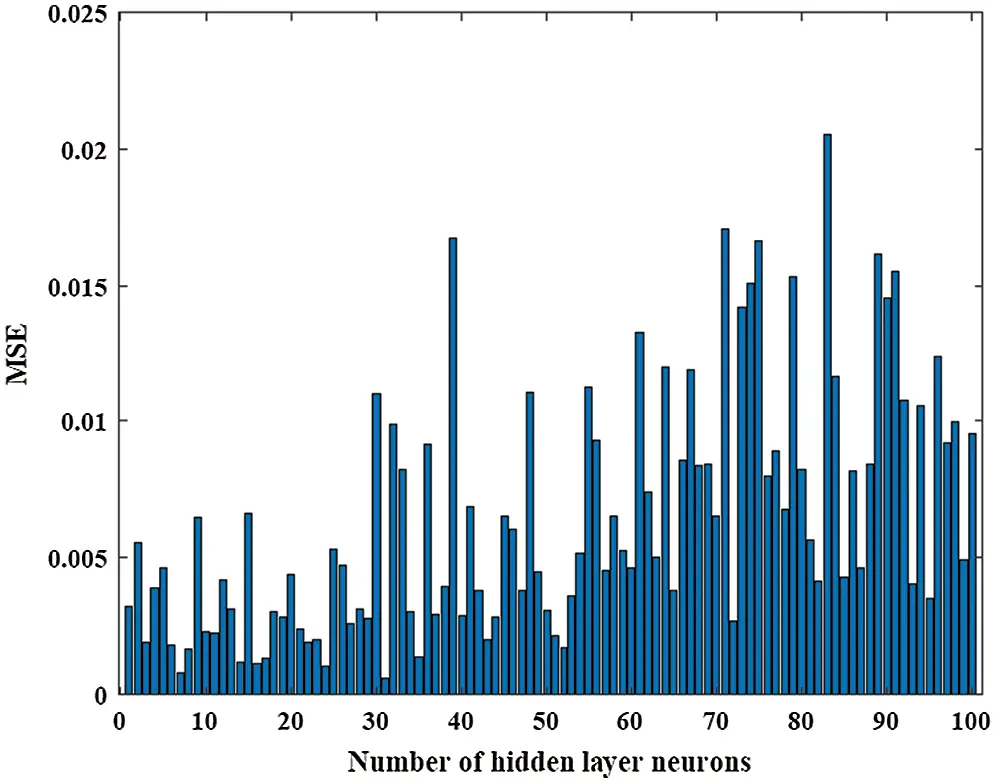Figure 7: Mean square error against the number of hidden layer neurons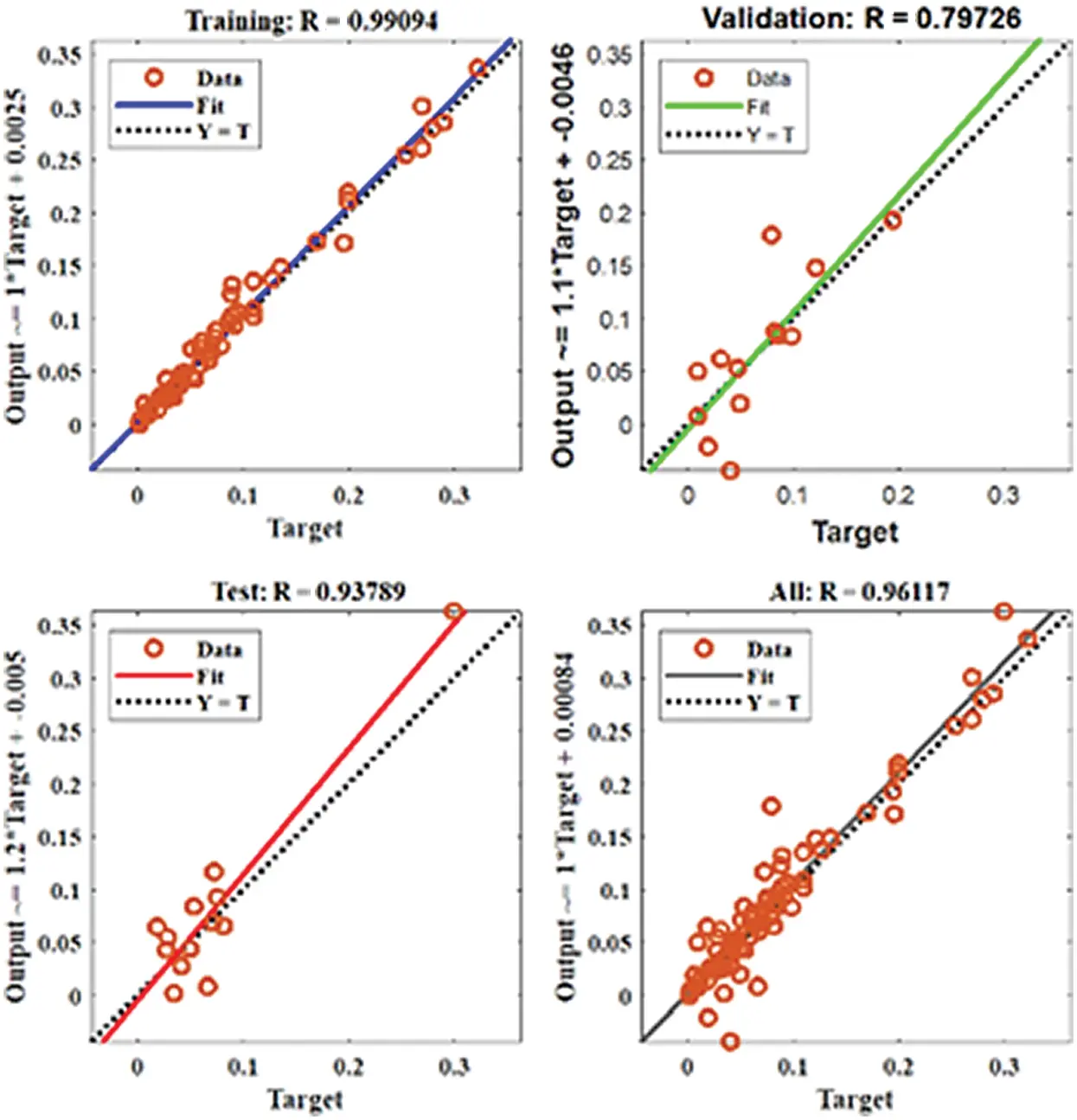Figure 8: Regression of the training, validation, testing, and overall dataset samples with 14 hidden layers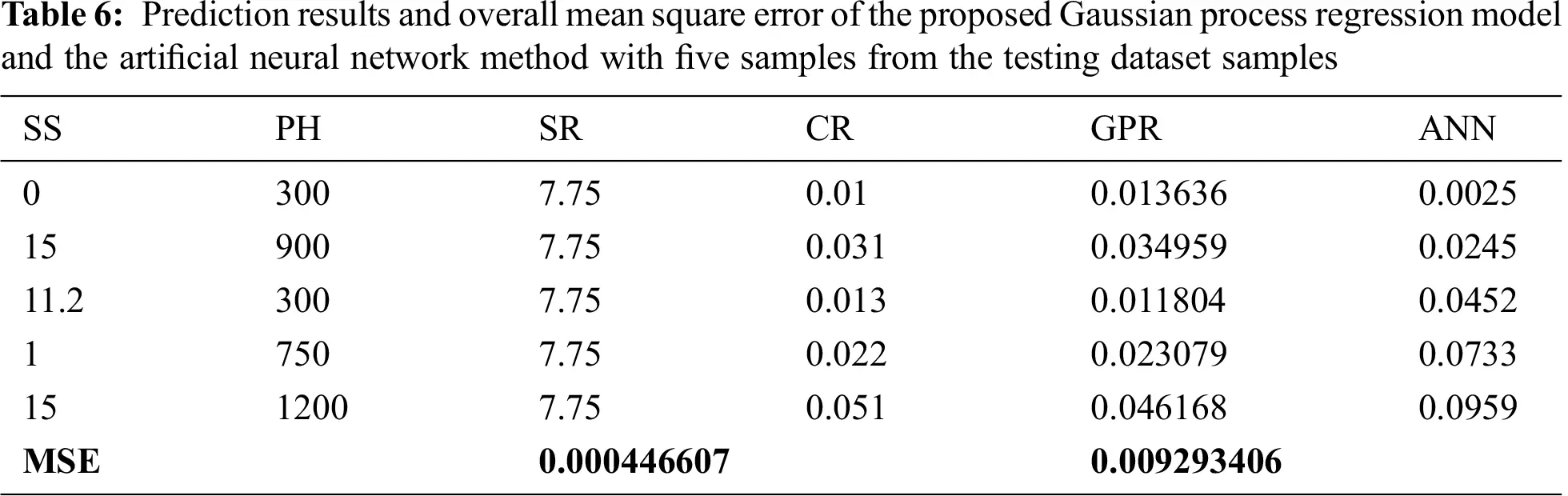5  Conclusions

The corrosion behavior of the Al–Si alloys in aqueous solutions was found to be dependent on Si content, solution pH, and solution stirring speed. Eutectic alloy (11.2% Si) showed the highest CR under different corrosion test conditions. Eutectic alloy presented a fine-grained structure with a high-energy structure. Thus, it had numerous galvanic corrosion areas. The addition of Si to pure Al as a hypoeutectic alloy (8% Si) or a hypereutectic alloy (15% Si) improved the CR of pure Al. The CR in simulated condensate solution with pH 3.5 was higher than that in other test solutions. The CR in the solution containing Al2O3 particles with pH 7.75 was very small compared with that of the solution containing H2SO4. In addition, the CR markedly increased with increasing stirring speed. Moreover, the Al–Si alloys and Al were attacked by pitting corrosion. The four MRLO methods, namely, DT, SVM, EN, and GPR, achieved good CR prediction accuracy. The four optimization regression methods were implemented and conducted in MATLAB/software regression toolbox. The optimal parameters of the four methods were determined using Bayesian optimization technique. GPR had the highest CR prediction accuracy with the lowest MSE of 0.000446607. The proposed GPR model was validated, and its effectiveness was compared with that of the ANN model. The overall MSE of the proposed GPR and ANN models for the testing dataset samples (21 samples) was 0.000446607 and 0.009293406, respectively. Under the conditions studied herein and by adopting the materials in this study, designers and process engineers can utilize the corrosion properties of the Al–Si alloys predicted by the four MRLO methods to save on costs, time, and experimental materials.

Funding Statement: The authors would like to acknowledge the financial support received from Taif University Researchers Supporting Project Number (TURSP-2020/61), Taif University, Taif, Saudi Arabia.

Conflicts of Interest: The authors declare that they have no conflicts of interest to report regarding the present study.

## References

1. I. Oztürk, G. H. Agaoglu, E. Erzi, D. Dispinar and G. Orhan, “Effects of strontium addition on the microstructure and corrosion behavior of A356 aluminum alloy,” Journal of Alloys and Compounds, vol. 763, no. 1–2, pp. 384–391, 2018.
2. Kh. Abd El-Aziz, D. Saber and H. E. M. Sallam, “Wear and Corrosion Behavior of Al-Si matrix composite reinforced with alumina,” Journal of Bio & Tribo-Corrosion, vol. 1, no. 1, pp. 1132, 2015.
3. M. Megahed, D. Saber and M. A. Agwa, “Modeling of wear behavior of Al-Si/Al2O3 metal matrix composites,” Physics of Metals and Metallography, vol. 120, no. 10, pp. 981–988, 2019.
4. M. Rosso, I. Peter, C. Castella and R. Molina, “Properties of AlZn10Si8Mg alloys for high performances application,” Light Metals, Cham, Switzerland, pp. 213–218,201
5. J. Šćepanović, V. Asanović and F. Bikić, “Mechanical properties and corrosion behaviour of Al-Si alloys for IC engine,” Journal of the Serbian Chemical Society, vol. 84, no. 5, pp. 503–516, 2019.
6. S. A. Salih, A. G. Gad, A. A. Mazhar and R. H. Tammam, “Effect of silicon alloying addition on corrosion behavior in some aqueous media,” Journal of Applied Electrochemistry, vol. 31, no. 10, pp. 1103–1108, 2001.
7. A. A. Mazhar, S. T. Arab and E. A. Noor, “The role of choloride ions and pH in the corrosion pitting of Al-Si alloys,” Journal of Applied Electrochemistry, vol. 31, no. 10, pp. 1131–1140, 2001.
8. A. A. Mazhar, S. T. Arab and E. A. Noor, “Electrochemical behavior of Al-Si alloys in acidic and alkaline media,” Bulletin of Electrochemistry, vol. 17, no. 10, pp. 449–458, 2001.
9. A. Dobkowsk, B. Adamczyk, J. Mizer, K. J. Kurzydłowski and A. Kiełbus, “The comparison of the microstructure and corrosion resistance of sand cast aluminum alloys,” Archives of Metallurgy and Materials, vol. 61, no. 1, pp. 209–212, 2016.
10. D. Saber, R. Abdel-Karim, A. A. Kandel and K. Abd El-Aziz, “Corrosive Wear of Alumina Particles Reinforced Al-Si Alloy Composites,” Physics of Metals and Metallography, vol. 121, no. 2, pp. 188–194, 2020.
11. M. K. Moatasem, “Use of artificial neural networks for prediction of mechanical properties of Al-Si alloys synthesized by stir casting,” Journal of Petroleum and Mining Engineering, vol. 21, no. 1, pp. 97–103, 2019.
12. X. Chen, Z. Yuan, Y. Zheng and W. Liu, “Prediction of carbon steel corrosion rate based on an alternating conditional expectation (ACE) algorithm,” Chemistry and Technology of Fuels and Oils, vol. 51, no. 6, pp. 728–739, 2016.
13. P. H. Thike, Z. Zhao, P. Liu, F. Bao, Y. Jin et al., “Based artificial neural network model for atmospheric corrosion prediction of carbon steel,” Computers, Materials & Continua, vol. 65, no. 3, pp. 2091–2109, 2020.
14. E. D. Kenny, R. S. C. Paredes, L. A. de Lacerda, Y. C. Sica, G. P. de Souza et al., “Artificial neural network corrosion modeling for metals in an equatorial climate,” Corrosion Science, vol. 51, no. 10, pp. 2266–2278, 2009.
15. M. Zhang, J. F. Yu, S. W. Yang and X. L. He, “Analysis in atmospheric corrosion behavior of bainite steel exposed in offshore platform based on the artificial neural network,” Advanced Materials Research, vol. 291–294, pp. 1212–1216, 2011.
16. C. M. Lo, Y. P. Chiu and M. D. Lin, “Predicting atmospheric corrosion rates of copper in Taiwan industrial zones using artificial neural network,” in IEEE Int. Conf. on Industrial Engineering and Engineering Management, 2017.
17. L. Li, P. Qiu, S. B. Xing and X. Su, “Research on corrosion rate prediction of aluminum alloys in typical domestic areas based on BP artificial neural network,” Advanced Materials Research, vol. 652, pp. 1088–1091, 2013.
18. R. Vera and S. Ossandón, “On the prediction of atmospheric corrosion of metals and alloys in Chile using artificial neural networks,” International Journal of Electrochemical Science, vol. 9, no. 12, pp. 7131–7151, 2014.
19. R. Willumeit, F. Feyerabend and N. Huber, “Magnesium degradation as determined by artificial neural networks,” Acta Biomaterialia, vol. 9, no. 10, pp. 8722–8729, 2013.
20. MathWorks, Accelerating the pace of engineering and science. © 1994-2021 The MathWorks, Inc.[Online].Available:https://ch.mathworks.com/help/stats/choose-regression-model-ptions.html.
21. S. Putatunda and K. Rama, “A Modified Bayesian Optimization based Hyper-Parameter Tuning Approach for Extreme Gradient Boosting,” in Fifteenth Int. Conf. on Information Processing (ICINPROpp. 1–6, December 2019.
22. W. William, B. Burank and P. Efstratios, “Hyperparameter optimization of machine learning models through parametric programming,” Computers and Chemical Engineering, vol. 139, pp. 1–12, 2020.
23. W. Jia, C. Xiu, Z. Hao, X. Li-Diong and D. Si-Hao, “Hyperparameter optimization for machine learnin models based on Bayesian optimization,” Journal of Electronic Science and Technology, vol. 17, pp. 26–40, 2019.
24. D. Saber, Kh. Abd El-Aziza, R. Abdel-Karim and A. A. Kandel, “Corrosive wear of alumina particles reinforced Al-Si alloy composites,” Physics of Metals and Metallography, vol. 121, no. 2, pp. 188–194, 2020.
25. N. d. Latona and P. Fetherston, “Wear-corrosion comparison of passivating vs nonpassivating alloys in a rated 3.5% aqueous solutions of sodium chloride,” Corrosion, vol. 57, no. 10, pp. 884–888, 2001.
26. S. S. M. Ghoneim, I. B. M. Taha and N. I. Elkalashy, “Integrated ANN-based proactive fault diagnostic scheme for power transformers using dissolved gas analysis,” IEEE Transactions on Dielectrics and Electrical Insulation, vol. 23, no. 3, pp. 1838–1845, 2016.
27. J.-H. Menke, N. Bornhorst and M. Brauna, “Distribution system monitoring for smart power grids with distributed generation using artificial neural networks,” International Journal of Electrical Power & Energy Systems, vol. 113, no. 4, pp. 472–480, 2019.
28. T. Ahmad, H. Chen and W. A. Shah, “Effective bulk energy consumption control and management for power utilities using artificial intelligence methods under conventional and renewable energy resources,” International Journal of Electrical Power & Energy Systems, vol. 109, no. 4, pp. 242–258, 2019.
29. S. Pintos, N. V. Queipode, O. T. Rincón and M. Morcillo, “Rincón and M.Morcillo Artificial neural network modeling of atmospheric corrosion in the MICAT project,” Corrosion Science, vol. 42, no. 1, pp. 35–52, 2000.
30. J. Cai, R. A. Cottis and S. B. Lyon, “Phenomenological modelling of atmospheric corrosion using an artificial neural network,” Corrosion Science, vol. 41, no. 10, pp. 2001–2030, 1999.This work is licensed under a Creative Commons Attribution 4.0 International License, which permits unrestricted use, distribution, and reproduction in any medium, provided the original work is properly cited.